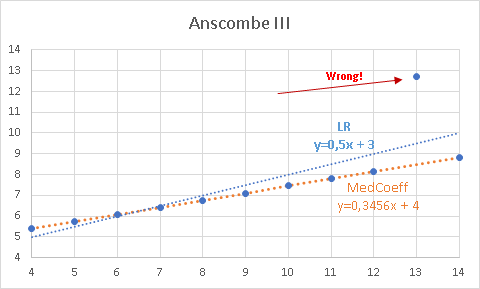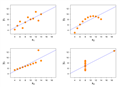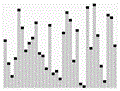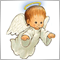# Self-regressive Median Coefficient - page 440442

Petr Nosek:

Thank you for your effort. Don't be sad I won't call you "Master of efficiency" :D

I really appreciate your approach. Even though you've failed you are still honest (unlike others).

I still have some difficulties to integrate that double arithmetic is so fast and efficient nowadays.

I don't care to fail or to be wrong, it's how we are learning. However I still have a feeling that it's possible to be optimized :-D726

Alain Verleyen:
Well that's not so simple. Considering a period of 32, for 129122 bars, there are 31*129122 coefficients, not 129121. (Neglecting the fact that older bars doesn't have enough data to be calculated correctly).
Can't the coefficients be stored?726

Petr Nosek:

Thank you for your effort. Don't be sad I won't call you "Master of efficiency" :D

I really appreciate your approach. Even though you've failed you are still honest (unlike others).

BTW I think that the better approach for OP's needs is using Linear Regression or Kalman filter or something similar.

https://en.wikipedia.org/wiki/Anscombe%27s_quartet

Example 3. Linear regression causes the slope to be 0.5. However, the point at x=12.74 is clearly wrong. So, if one takes all possible coefficients and take the median out of them, gets the orange line (median slope of 0.3456). This is the simplest case of quantile regression. In a practical way. :P

So there's no chance of using mean (LR uses it) or any other calculation based on it to predict the future. And all calculations based on the mean will fail. People forgot that descriptive statistics is used to compare different known datasets.Anscombe's quartet - Wikipedia
• en.wikipedia.org
Anscombe's quartet comprises four datasets that have nearly identical simple descriptive statistics, yet appear very different when graphed. Each dataset consists of eleven (x,y) points. They were constructed in 1973 by the statistician Francis Anscombe to demonstrate both the importance of graphing data before analyzing it and the effect of...177528

Alain Verleyen:

I still have some difficulties to integrate that double arithmetic is so fast and efficient nowadays.

I don't care to fail or to be wrong, it's how we are learning. However I still have a feeling that it's possible to be optimized :-D

Had some ideas (to eliminate some elements - and that would largely make the sorting faster too), but since the decisive is the price (regardless of the rest), we can not "predict" where the median will fall without calculation, and that brings us back to square one

One of those :)726

Had some ideas (to eliminate some elements - and that would largely make the sorting faster too), but since the decisive is the price (regardless of the rest), we can not "predict" where the median will fall without calculation, and that brings us back to square one

One of those :)

Median of medians can optimise MathMedian.177528

I was experimenting more something like this :

```//------------------------------------------------------------------
//------------------------------------------------------------------
#property indicator_separate_window
#property indicator_buffers 6
#property indicator_plots   2
#property indicator_label1  "Test value"
#property indicator_type1   DRAW_FILLING
#property indicator_color1  clrHotPink,clrLightGreen
#property indicator_label2  "Test value"
#property indicator_type2   DRAW_COLOR_LINE
#property indicator_color2  clrCrimson,clrLimeGreen
#property indicator_width2  2

input int                inpPeriod = 15;          // Period
input ENUM_APPLIED_PRICE inpPrice  = PRICE_CLOSE; // Price
double val[],valc[],prices[],fillu[],filld[],momentums[];
//------------------------------------------------------------------
//
//------------------------------------------------------------------
int OnInit()
{
IndicatorSetInteger(INDICATOR_DIGITS,8);
SetIndexBuffer(0,fillu,INDICATOR_DATA);
SetIndexBuffer(1,filld,INDICATOR_DATA);
SetIndexBuffer(2,val,INDICATOR_DATA);
SetIndexBuffer(3,valc,INDICATOR_COLOR_INDEX);
SetIndexBuffer(4,prices,INDICATOR_CALCULATIONS);
SetIndexBuffer(5,momentums,INDICATOR_CALCULATIONS);
PlotIndexSetInteger(0,PLOT_SHOW_DATA,false);
IndicatorSetString(INDICATOR_SHORTNAME,"Self-regressive Median Coefficient test ("+(string)inpPeriod+")");
return(INIT_SUCCEEDED);
}
void OnDeinit(const int reason) { return; }

//------------------------------------------------------------------
//
//------------------------------------------------------------------
double CoeffBuffer[];
int OnCalculate(const int rates_total,
const int prev_calculated,
const datetime& time[],
const double& open[],
const double& high[],
const double& low[],
const double& close[],
const long& tick_volume[],
const long& volume[],
{
if (Bars(_Symbol,_Period)<rates_total) return(0);

//
//---
//

ArrayResize(CoeffBuffer,inpPeriod);
for (int i=(int)MathMax(prev_calculated-1,0); i<rates_total && !_StopFlag; i++)
{
prices[i] = getPrice(inpPrice,open,close,high,low,i,rates_total);
momentums[i] = 0;
for (int k=1; k<=inpPeriod && (i-k)>=0; k++) momentums[i] += prices[i]-prices[i-k];
ArrayCopy(CoeffBuffer,momentums,0,i-inpPeriod+1,inpPeriod);
ArraySort(CoeffBuffer);
val[i]    = (inpPeriod%2==1) ? (CoeffBuffer[inpPeriod/2]) : (0.5*(CoeffBuffer[(inpPeriod-1)/2]+CoeffBuffer[(inpPeriod+1)/2]));
valc[i]   = (val[i]>0) ? 1 : 0;
fillu[i]  = 0;
filld[i]  = val[i];
}
return(rates_total);
}

//------------------------------------------------------------------
//
//------------------------------------------------------------------
double getPrice(ENUM_APPLIED_PRICE tprice,const double &open[],const double &close[],const double &high[],const double &low[],int i,int _bars)
{
if (i>=0)
switch(tprice)
{
case PRICE_CLOSE:     return(close[i]);
case PRICE_OPEN:      return(open[i]);
case PRICE_HIGH:      return(high[i]);
case PRICE_LOW:       return(low[i]);
case PRICE_MEDIAN:    return((high[i]+low[i])/2.0);
case PRICE_TYPICAL:   return((high[i]+low[i]+close[i])/3.0);
case PRICE_WEIGHTED:  return((high[i]+low[i]+close[i]+close[i])/4.0);
}
return(0);
}```

But, as obvious, the results are *regardless of the fact that they are sometimes interesting) just approximations (compared to "full calculation")726

Alain Verleyen:

So at my surprise, I didn't succeed. The main optimization idea I had was to reduce the number of division operations needed :

As this operation is done in a loop for every candle i, there are a lot of repetitions of the exact same operation. So the idea was to do all the operations once and to memorize them (see attached how I did). However it doesn't improve this speed, even while the numbers of operations was reduced by a factor 16 !

From 64 millions to 4 millions division operations, but no change in execution time. I didn't expect that. That means double arithmetic CPU is very efficient and cached very well all the results.

Also, though this

Alain Verleyen:

So at my surprise, I didn't succeed. The main optimization idea I had was to reduce the number of division operations needed :

As this operation is done in a loop for every candle i, there are a lot of repetitions of the exact same operation. So the idea was to do all the operations once and to memorize them (see attached how I did). However it doesn't improve this speed, even while the numbers of operations was reduced by a factor 16 !

From 64 millions to 4 millions division operations, but no change in execution time. I didn't expect that. That means double arithmetic CPU is very efficient and cached very well all the results.

Also, though this imbricated loop with division operations is time consuming, the main bottleneck in the ArraySort(), the speed impact is more than 3 times the one of the loops. So even if the "division" optimization had worked that global impact would have been low (~20% max).

That was an interesting exercise, even if it failed.

Attached the code (as we are on week-end I didn't pay attention to "live" update).

loop with division operations is time consuming, the main bottleneck in the ArraySort(), the speed impact is more than 3 times the one of the loops. So even if the "division" optimization had worked that global impact would have been low (~20% max).

That was an interesting exercise, even if it failed.

Attached the code (as we are on week-end I didn't pay attention to "live" update).

Does MQL 5 quickselect implemented? If not, how can I contact one of the developers to include it?Quickselect - Wikipedia
• en.wikipedia.org
Quickselect Class Data structure Worst-case performance Best-case performance Average performance In computer science, quickselect is a selection algorithm to find the kth smallest element in an unordered list. It is related to the quicksort sorting algorithm. Like quicksort, it was developed by Tony Hoare, and thus is also known as Hoare's...726

Selection sort can find the median. However, I believe it is slower than ArraySort(), which uses quicksort. Did not test.

```double selection(double &array[], int &index)
{
int i, j, position, size = ArraySize(array);
double swap;

for (i = 0; i <= index; i++)
{
position = i;
for (j = i+1; j < size; j++)
{
if (array[j] < array[position])
position = j;

// swap the found minimum element with the first element
swap = array[position];
array[position] = array[i];
array[i] = swap;
}

}
return(array[index]);
}```3558

Arthur Albano:

https://en.wikipedia.org/wiki/Anscombe%27s_quartet

Example 3. Linear regression causes the slope to be 0.5. However, the point at x=12.74 is clearly wrong. So, if one takes all possible coefficients and take the median out of them, gets the orange line (median slope of 0.3456). This is the simplest case of quantile regression. In a practical way. :P

So there's no chance of using mean (LR uses it) or any other calculation based on it to predict the future. And all calculations based on the mean will fail. People forgot that descriptive statistics is used to compare different known datasets.

It depends on kind of dataset. Your example is not similar to financial market dataset much. First example from your link passes better to our purpose IMHO. In this case could be linear regression better choice. But we shouldn't forget we still talk about forecasting unpredictable future ;-)726

Implementation of quickselect. But there's something wrong...

```#define SWAP(a, b) a ^= (b ^= (a ^= b));
#define SORT(a, b, c) {if(a > b) SWAP(a,b); if(a > c) SWAP(a,c); if (b>c) SWAP(b,c)}

// Partition using Lomuto partition scheme

int partition(int &a[], int left, int right, int pivotIndex)

{
// Pick pivotIndex as pivot from the array
int pivot = a[pivotIndex];

// Move pivot to end
SWAP(a[pivotIndex], a[right]);

// elements less than pivot will be pushed to the left of pIndex
// elements more than pivot will be pushed to the right of pIndex
// equal elements can go either way
int pIndex = left;
int i;

// each time we finds an element less than or equal to pivot, pIndex
// is incremented and that element would be placed before the pivot.
for (i = left; i < right; i++)
{
if (a[i] <= pivot)
{
SWAP(a[i], a[pIndex]);
pIndex++;
}
}

// Move pivot to its final place
SWAP(a[pIndex], a[right]);

// return pIndex (index of pivot element)

return pIndex;

}

// Returns the k-th smallest element of list within left..right

// (i.e. left <= k <= right). The search space within the array is

// changing for each round - but the list is still the same size.

// Thus, k does not need to be updated with each round.

int quickselect(int &A[], int left, int right, int k)

{
// If the array contains only one element, return that element
if (left == right)
return A[left];

// select a pivotIndex between left and right

int pivotIndex = left + rand() % (right - left + 1);

pivotIndex = partition(A, left, right, pivotIndex);

// The pivot is in its final sorted position
if (k == pivotIndex)
return A[k];

// if k is less than the pivot index

else if (k < pivotIndex)

return quickselect(A, left, pivotIndex - 1, k);

// if k is more than the pivot index

else
return quickselect(A, pivotIndex + 1, right, k);

}

void OnStart()
{

int A[] = { 7, 4, 6, 3, 9, 1 };

int size = ArraySize(A);
int medianRank = (size-1) / 2;

int med = quickselect(A,0,size-1,medianRank);

return;
}```